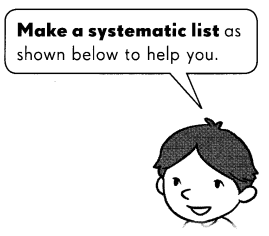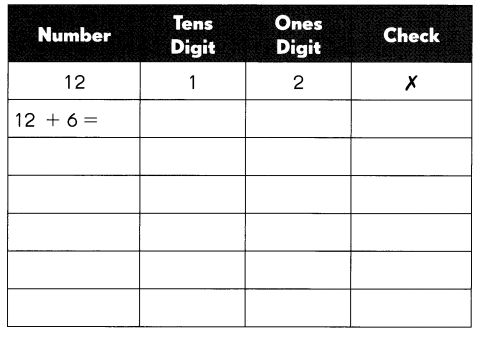# Math in Focus Grade 3 Chapter 6 Answer Key Multiplication Tables of 6, 7, 8, and 9

Go through the Math in Focus Grade 3 Workbook Answer Key Chapter 6 Multiplication Tables of 6, 7, 8, and 9 to finish your assignments.

## Math in Focus Grade 3 Chapter 6 Answer Key Multiplication Tables of 6, 7, 8, and 9

Math Journal

Question 1.
Show 3 × 6 on the number line.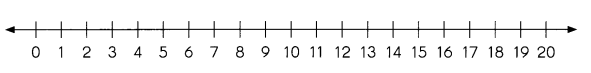3 × 6 = 18.

Explanation:
The number line of 3 × 6 is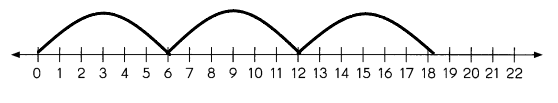Question 2.
Draw an array model to show 5 × 7.
The array model of 5 × 7 is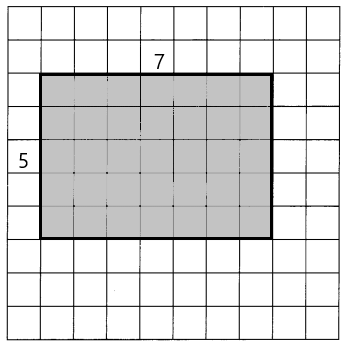Question 3.
Show 8 × 9 with the area model.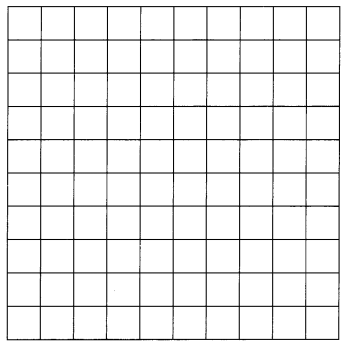The model of 8 × 9 is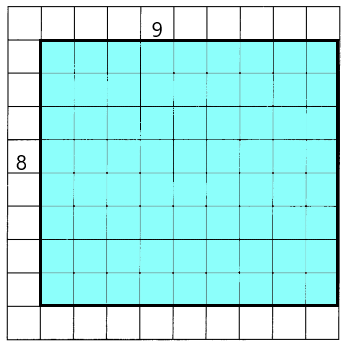Challenging Practice

Complete each skip-counting pattern.

Question 1.
70 63 56 ____ ___ 35
___ ____ 14 7
70, 63, 56, 49, 42, 35, 28, 21, 14, 7.

Explanation:
As we can see that the digits are multiplies of 7, so the multiplies are 70, 63, 56, 49, 42, 35, 28, 21, 14, 7.

Question 2.
80 72 64 ___ ____ ____
___ ____ 16 8
80, 72, 64, 56, 48, 40, 32, 24, 16, 8.

Explanation:
As we can see that the digits are multiplies of 8, so the multiplies are 80, 72, 64, 56, 48, 40, 32, 24, 16, 8.

Identify a number that does not belong in the group. Then write the reason. Use the number patterns to help you.

Question 3.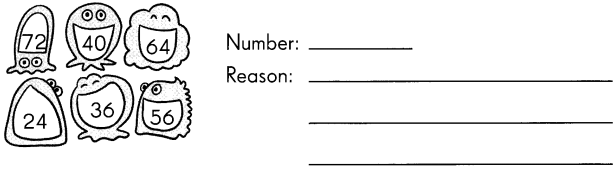36.

Explanation:
The given numbers are multiples of 8 and in that 36 is not a multiple of 8. So 36 doesn’t belong to this group.

Question 4.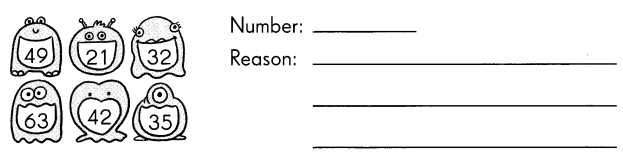32.

Explanation:
The given numbers are multiples of 7 and in that 32 is not a multiple of 7. So 32 doesn’t belong to this group.